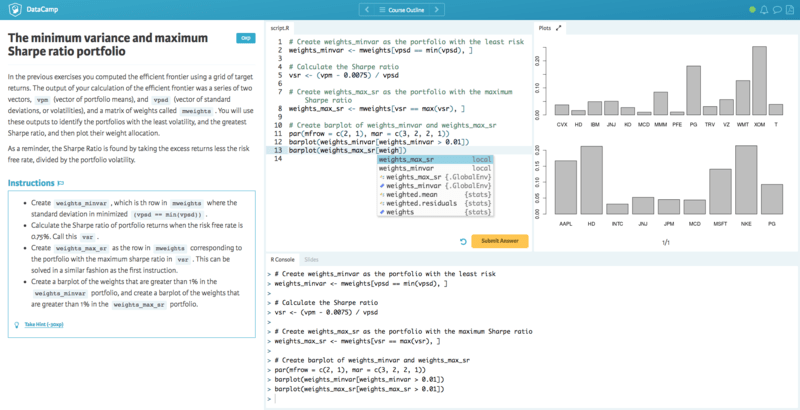Want to share your content on R-bloggers? click here if you have a blog, or here if you don't.

### New Course Announcement: Introduction to Portfolio Analysis in R

One golden rule in investing is to always test your portfolio strategy on historical data. Then, you should constantly monitor the strategy’s performance after you begin trading. In this course, you will learn tools to critically analyzing portfolio returns using the R package PerformanceAnalytics. The course also shows how to estimate the portfolio weights that optimally balance risk and return. This is a data-driven tutorial that combines portfolio theory with practical applications in R, illustrated on real-life examples of equity portfolios and asset allocation problems. Start the course for free now!### Course Breakdown

Chapter 1: The building blocks

Asset returns and portfolio weights; those are the building blocks of a portfolio return. This chapter is about computing those portfolio weights and returns in R.

Chapter 2: Analyzing performance

The history of portfolio returns reveals valuable information about how much the investor can expect to gain or lose. This chapter introduces the R functionality to analyze the investment performance based on a statistical analysis of the portfolio returns. It includes graphical analysis and the calculation of performance statistics expressing average return, risk and risk-adjusted return over rolling estimation samples.

Chapter 3: Performance drivers

In addition to studying portfolio performance based on the observed portfolio return series, it is relevant to find out how individual (expected) returns, volatilities and correlations interact to determine the total portfolio performance.

Chapter 4: Optimizing the portfolio

We have up to now considered the portfolio weights as given. In this chapter, you learn how to determine in R the portfolio weights that are optimal in terms of achieving a target return with minimum variance while satisfying constraints on the portfolio weights.